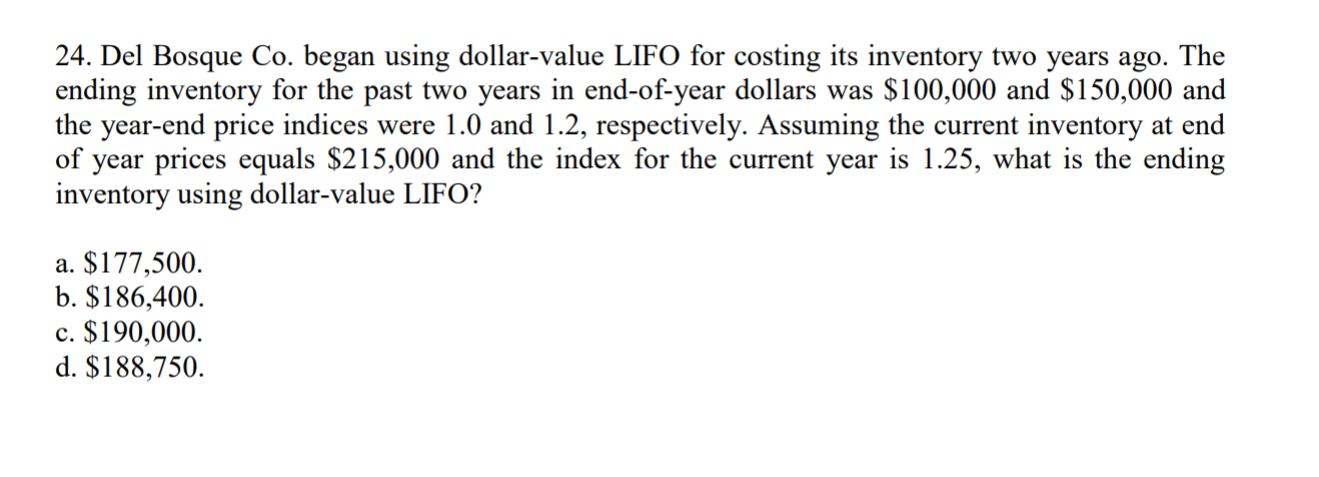# 24. Del Bosque Co. began using dollar-value LIFO for costing its inventory two years ago. Theending inventory for the past two years in end-of-year dollars was S100,000 and S150,000 andthe year-end price indices were 1.0 and 1.2, respectively. Assuming the current İnventory at endof year prices equals \$215,000 and the index for the current year is 1.25, what is the endinginventory using dollar-value LIFO?a. \$177,500b. \$186,400.c. \$190,000.d. \$188,750.

Question
21 viewshelp_outlineImage Transcriptionclose24. Del Bosque Co. began using dollar-value LIFO for costing its inventory two years ago. The ending inventory for the past two years in end-of-year dollars was S100,000 and S150,000 and the year-end price indices were 1.0 and 1.2, respectively. Assuming the current İnventory at end of year prices equals \$215,000 and the index for the current year is 1.25, what is the ending inventory using dollar-value LIFO? a. \$177,500 b. \$186,400. c. \$190,000. d. \$188,750. fullscreen
check_circle

Step 1

Step1: Calculating the value of ending inventory at base year price. We have,

Ending inventory at base year price = Ending inventory / Current year price index

Ending inventory at base year price = \$ 215,000 / 1.25

Ending inventory at base year price = \$ 172,000

Therefore, the ending inventory at base year price shall be \$ 172,000

Step 2

Step2: Calculating the value of real-dollar quantity increase in inventory. We have,

Real-dollar quantity increase in inventory = Ending inventory at base year price – Base year                                      inventory

Real-dollar quantity increase in inventory = \$ 172,000 - \$ 100,000

Real-dollar quantity increase in inventory = \$ 72,000

Therefore, the real-dollar quantity increase in inventory shall be \$ 72,000

Step 3

Step3: Calculating the value of real dollar increase in inventory at year-end-prices. We have,

Value of real dollar increase in inventory at year-end-prices = Real-dollar quantity increase in inventory x Curre...

### Want to see the full answer?

See Solution

#### Want to see this answer and more?

Solutions are written by subject experts who are available 24/7. Questions are typically answered within 1 hour.*

See Solution
*Response times may vary by subject and question.
Tagged in